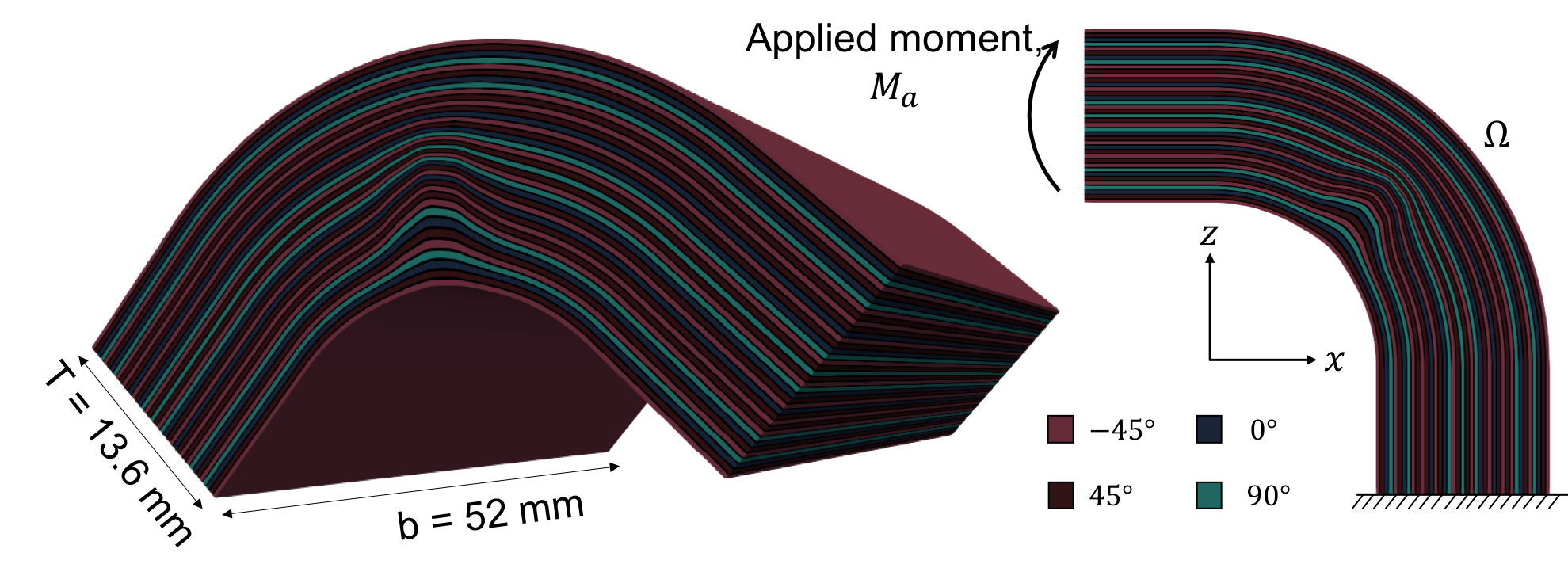# Composite material with random wrinkle¶

## Overview¶

This model implements the 3D anisotropic linear elasticity equations for a composite part with randomised wrinkle. The maximum deflection of the part is estimated by embedding a wrinkle into a high fidelity FE simulation using the high performance toolbox dune-composites.Anne Reinarz

## Run¶

docker run -it -p 4242:4242 linusseelinger/model-dune-composites:latest


## Properties¶

Model

Description

forward

Linear elasticity

### forward¶

Mapping

Dimensions

Description

input



Coefficients of a Karhunen Loeve expansion of a wrinkle.

output



Maximum deflection of the composite part.

Feature

Supported

Evaluate

True

False

ApplyJacobian

False

ApplyHessian

False

Config

Type

Default

Description

ranks

int

2

Number of MPI ranks (i.e. parallel processes) to be used.

stack

string

“example2.csv”

Path to the stacking sequence to be run.

Mount directory

Purpose

None

## Source code¶

Model sources here.

## Description¶

In the simulation the composite strength of a corner part with a wrinkle is modeled and the maximum deflection is returned. The stacking sequence of the composite corner part can be set using the “stack” configuration parameter, by default a 12 layer setup is used. A simplified model for a 3D bending test was used. The curved composite parts were modelled with shortened limbs of length 10 mm. A unit moment was applied to the end of one limb using a multi-point constraint, with homogeneous Dirichlet conditions applied at the end of the opposite limb. This gives the same stress field towards the apex of the curved section as a full 3D bending test. The analysis assumes standard anisotropic 3D linear elasticity and further details on the numerical model and discretisation can be found in .

The wrinkle defect is defined by a deformation field $$W:\Omega \rightarrow \mathbb R^3$$ mapping a composite component from a pristine state to the defected state.

The wrinkles are defined by the wrinkle functions

$W(x,\xi) = g_1(x_1)g_3(x_3)\sum_{i=1}^{N_w} a_i f_i(x_1,\lambda),$

where $$g_i(x_i)$$ are decay functions , $$f_i(x_1,\lambda)$$ are the first $$N_w$$ Karhunen-Lo’{e}ve (KL) modes parameterized by the length scale $$\lambda$$ and $$a_i$$ the amplitudes. The amplitude modes and the length scale can be taken as random variables, so that the stochastic vector is defined by $$\boldsymbol \xi = [a_1,a_2,\ldots,a_{N_w},\lambda]^T$$. The wrinkles are prismatic in $$x_2$$, e.g. the wrinkle function is assumed to have no $$x_2$$ dependency. For more details on the wrinkle representation see .